Refer to our Texas Go Math Grade 8 Answer Key Pdf to score good marks in the exams. Test yourself by practicing the problems from Texas Go Math Grade 8 Module 9 Quiz Answer Key.

9.1 Volume of Cylinders

Find the volume of each cylinder. Round your answers to the nearest tenth If necessary. Use 3.14 for π.

Question 1.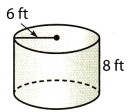Height of cylinder = 8 ft
Volume of cylinder = πr2h
Volume = 3.14 × 62 × 8
Volume = 3.14 × 36 × 8
Volume = 904.32 ft3

Question 2.
A can of juice has a radius of 4 inches and a height of 7 inches. What is the 8ft volume of the can?
Radius if cyLindrical can = 4 in
Height of cylindrical can = 7 in
Volume of cylinder = πr2h
Volume = 3.14 × 42 × 7
Volume = 351.68 in3
Volume ≈ 351.7 in3

9.2 Volume of Cones

Find the volume of each cone. Round your answers to the nearest tenth if necessary. Use 3.14 for π.

Question 3.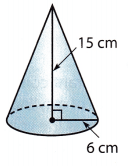Radius of base of cone = 6 cm
Height of cone = 15 cm
Volume of cone = $$\frac{1}{3}$$πr2h
Volume = $$\frac{1}{3}$$ × 3.14 × 62 × 15
Volume = 565.2 cm3

Question 4.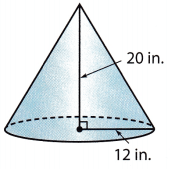Radius of base of cone = 12 in
Height of cone = 20 in
Volume of cone = $$\frac{1}{3}$$πr2h
Volume = $$\frac{1}{3}$$ × 3.14 × 122 × 20
Volume = 3014.4 cm3

9.3 Volume of Spheres

Find the volume of each sphere. Round your answers to the nearest tenth if necessary. Use 3.14 for π.

Question 5.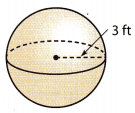Radius of sphere = 3 ft
Volume of sphere = $$\frac{4}{3}$$πr3
Volume = $$\frac{4}{3}$$ × 3.14 × 33
Volume = 113.04 ft3
Volume ≈ 113 ft3

Question 6.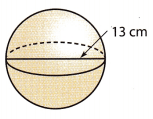Diameter of a sphere = 13 cm
Radius of the sphere = $$\frac{13}{2}$$ = 6.5 cm
Volume of the sphere = $$\frac{4}{3}$$πr3
Volume of the sphere = $$\frac{4}{3}$$ × 3.14 × 6.53
Volume of the sphere = 1149.7633 cm3
Volume ≈ 1149.8 cm3

Essential Question

Question 7.
What measurements do you need to know to find the volume of a cylinder? a cone? a sphere?
Sphere: For finding the volume of the sphere, the radius is to be measured.
Volume of sphere = $$\frac{4}{3}$$πr3

Cylinder : To calculate the volume of cylinder, we need to find out the base radius of base of the cylinder along with the height of the cylinder.
Volume of cylinder = πr2h

Cone : To calculate the volume of cone, we need to measure the base radius of the base of the cone along with the height of the cone.
Volume of cone = $$\frac{1}{3}$$πr2h

Texas Go Math Grade 8 Module 9 Mixed Review Texas Test Prep Answer Key

Selected Response

Question 1.
The bed of a long bed pickup truck measures 4 feet by 8 feet. Which is the length of the longest thin metal bar that will lie flat in the bed?
(A) 11 ft 3 in.
(B) 10 ft. 0 in.
(C) 8 ft 11 in.
(D) 8 ft 9 in.
(D) 8 ft 9 in.

Explanation:
The length of the longest thin metal bar that will lie flat in the bed is equal to the length of the bed’s hypotenuse. Let a = 4 and b = 8. Using the Pythagorean Theorem, we have:
a2 + b2 = c2
42 + 82 = c2
16 + 64 = c2
80 = c2
Rounding the length of the hypotenuse to the nearest tenth of a foot, we get:
c ≈ 8.9ft.
Therefore, the length of the longest thin metal bar that will lie flat in the bed is 8ft 9 in.

Question 2.
Using 3.14 for π, what is the volume of the cylinder below to the nearest tenth?(A) 102 cubic yards
(B) 347.6 cubic yards
(C) 1,091.6 cubic yards
(D) 4,366.4 cubic yards
(C) 1,091.6 cubic yards

Explanation:
Base diameter 11.4 yd
Base radius r = $$\frac{11.4}{2}$$
Base radius r = 5.7 yd
Height = 10.7 yd
Volume of a cylinder = πr2h
V = 3.14 × 5.72 × 10.7
V = 1091.59902 yd3
V ≈ 1091.6 yd3

Question 3.
Rhett made mini waffle cones for a birthday party. Each waffle cone was 3.5 inches high and had a radius of 0.8 inches. What is the volume of each cone to the nearest hundredth?
(A) 1.70 cubic inches
(B) 2.24 cubic inches
(C) 2.34 cubic inches
(D) 8.79 cubic inches
(C) 2.34 cubic inches

Explanation:
Base radius r = 0.8 in
Height = 3.5 in
Volume of a cone = $$\frac{1}{3}$$πr2h
V = $$\frac{1}{3}$$ × 3.14 × 0.82 × 3.5
V = 2.3445 in3
V ≈ 2.34 in3

Question 4.
Using 3.14 for π, what is the volume to the nearest tenth of a cone that has a height of 17 meters and a base with a radius of 6 meters?
(A) 204 cubic meters
(B) 640.6 cubic meters
(C) 2,562.2 cubic meters
(D) 10,249 cubic meters
(B) 640.6 cubic meters

Explanation:
Find the volume of a cone that has a height of 17 meters and a base with a radius of 6 meters.
Use the formula for the volume of a cone: $$\frac{1}{3}$$πr2h
Base radius r = 6 m
Height = 17 m
Volume of a cone = $$\frac{1}{3}$$πr2h
V = $$\frac{1}{3}$$ × 3.14 × 62 × 17
V = 640.56 m3
V ≈ 640.6 m3

Question 5.
Using 3.14 for π, what is the volume of the sphere to the nearest tenth?(A) 4180 cubic centimeters
(B) 5572.5 cubic centimeters
(C) 33,434.7 cubic centimeters
(D) 44,579.6 cubic centimeters
(B) 5572.5 cubic centimeters

Explanation:
Diameter of the sphere = 22 cm
Radius r = $$\frac{22}{2}$$ cm
Volume of sphere = $$\frac{4}{3}$$πr3
Volume = $$\frac{4}{3}$$ × 3.14 × 113
Volume = 5572.4533 cm3
Volume ≈ 5572.5 cm3

Gridded Response

Question 6.
A diagram of a deodorant container is shown. It is made up of a cylinder and half of a sphere. What is the volume of the whole container to the nearest tenth cubic centimeter? Use 3.14 for π.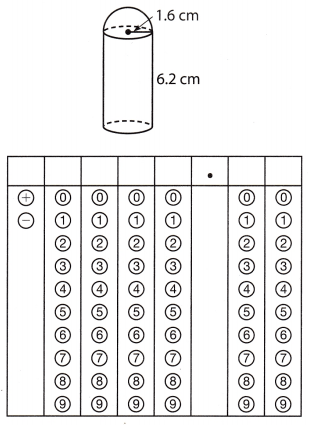a) Radius of a cylinder as well as the half sphere = 1.6 cm
Height = 6.2 cm
Volume of the half sphere = $$\frac{2}{3}$$πr3
Volume of the half sphere = $$\frac{2}{3}$$ × 3.14 × 1.63
Volume of the half sphere = 8.574 cm3

b) Volume of the cylinder = πr2h
Volume of the cylinder = 3.14 × 1.62 × 6.2
Volume of the cylinder = 49.838 cm3

c) Volume of the whole figure = Volume of the half sphere + Volume of the cylinder
Volume of the whole figure = 8.5 74 + 49.838
Volume of the whole figure = 58.4 12 cm3
Volume of the whole figure ≈ 58.4 cm3

a) Volume of the half sphere ≈ 8.574 cm3
b) Volume of the cylinder ≈ 49.838 cm3
c) Volume of the whole figure ≈ 58.4 cm3

Scroll to Top